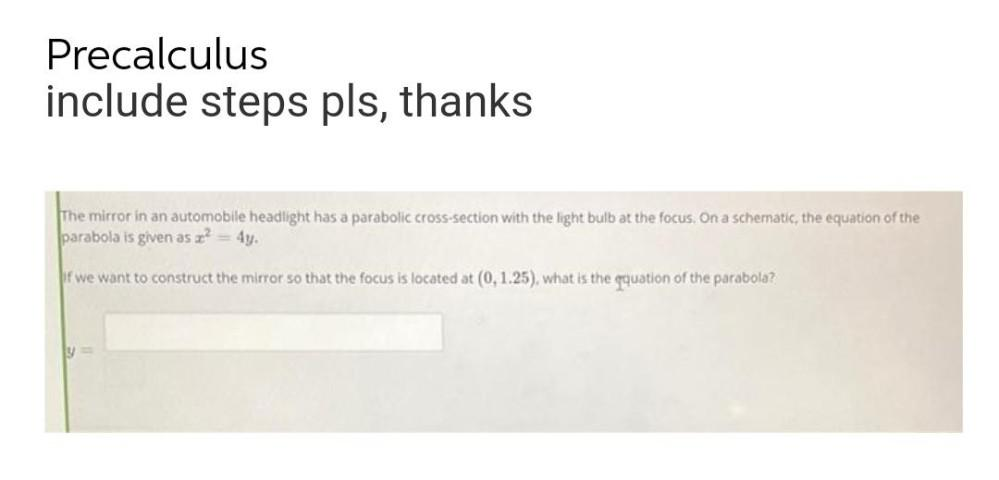Question:

# The mirror in an automobile headlight has a parabolic cross-section with the light bulb at the focus. On a schematic, the equation of the parabola is given as x^2= 4y. if we want to construct the mirrThe mirror in an automobile headlight has a parabolic cross-section with the light bulb at the focus. On a schematic, the equation of the parabola is given as x^2= 4y. if we want to construct the mirror so that the focus is located at (0,1.25), what is the equation of the parabola?